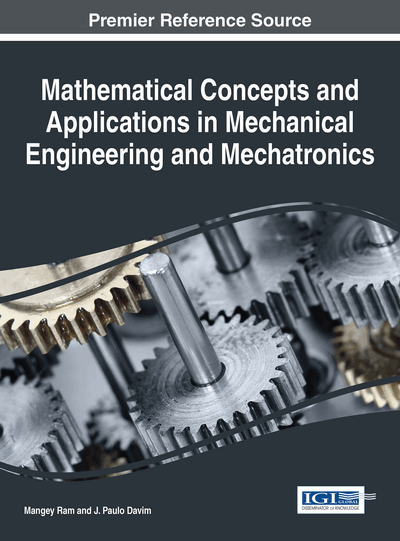# Development of a Material Constitutive Model and Simulation Technique to Predict Nonlinearities in Piezoelectric Materials under Weak Electric Fields

M. K. Samal (Bhabha Atomic Research Centre, India & Homi Bhabha National Institute, India)
DOI: 10.4018/978-1-5225-1639-2.ch013

## Abstract

Piezoceramic materials exhibit different types of nonlinearities depending upon the magnitude of the mechanical and electric field strength in the continuum. Some of the nonlinearities observed under weak electric fields are: presence of superharmonics in the response spectra and jump phenomena etc. especially if the system is excited near resonance. It has also been observed by many researchers that, at weak alternating stress fields, the relationship between the piezoelectrically induced charge and applied stress in ferroelectric ceramics, has the same form as the Rayleigh law (for magnetization versus magnetic field) in ferromagnetic materials. Applicability of the Rayleigh law to the piezoelectric effect has been demonstrated for Lead Zirconate Titanate ceramics by many researchers and their experimental results indicate that the dominant mechanism responsible for piezoelectric hysteresis and the dependence of the piezoelectric coefficient on the applied alternating stress is the pinning of non-180° domain walls. In this chapter, the Rayleigh law for ferromagnetic hysteresis has been modified and incorporated in a nonlinear electric enthalpy function and then applied in the analysis of hysteresis behavior of piezoelectric continua. Analytical solutions have been derived for a cantilever beam actuated by two piezo-patches attached to the top and bottom of the beam and excited by opposite electric fields. Analysis has been carried out at different electric field excitations of varying amplitude and frequencies and the results have been compared with the available experimental results from literature.
Chapter Preview
Top

## Introduction

Piezoceramic materials find wide applications in many different areas of modern technology (e.g. aerospace structures) and their use is increasing day by day (Crawley, 1994). Prediction of performance of the structures with piezoelectric sensors and actuators (for various control applications such as active shape, vibration and noise control etc.) requires thorough understanding of the piezoelectric material behaviour at different applied electrical and mechanical fields. Though nonlinearities under strong electric fields such as dielectric and butterfly hysteresis have drawn attention of many authors in recent times (Viswamurthy et al., 2007; Viswamurthy & Ganguli, 2007; Hwang & McMeeking, 1998; Thakkar & Ganguli, 2004, 2006, 2007), modeling nonlinear behaviour of piezoceramics under weak electric fields is limited in literature.

With a number of piezoelectric finite element (FE) models existing in the literature to cope with complicated boundary problems of smart structures, it is very important to develop a simple procedure to obtain analytical solutions for a few simple geometries for verification of finite element formulations and also to gain confidence on the procedure. Moreover it is also necessary to develop analytical solutions to determine the various material parameters including those of the nonlinear model to be used in the finite element analysis. The coupling of mechanical and electrical properties of piezoelectric materials makes the analytical solution complicated. Piezoelectric plates composed of orthotropic or anisotropic layers are usually analyzed using laminated composite plate theory. For the analytical solution of these plates, Pagano (1970) first presented the exact solution of a laminated plate under cylindrical bending. Ray et al. (1992, 1993a, b, 1998), Heyliger and Brooks (1996) extended this methodology to develop the exact solutions for the piezoelectric laminated plates with the help of Eshelby-Stroh formalism. Later, taking the thermal effect into account, Xu et al. (1995) presented a 3-D analysis for the coupled thermo-electro-elastic response of multilayered hybrid composite plates with four simply supported edges by using the state space method. Dube et al. (1996) developed a series solution method for a single layer thermo-electro-elastic plate subjected to cylindrical bending using the approach of Pagano (1970).

To solve the dynamic problem of piezoelectric array elements, several simplified solutions for modeling the linear piezoelectric behaviour in 1-D, 2-D and 3-D domains were tried. Ray et al. (1998) presented an exact solution for analyzing dynamics of composite plates with piezoelectric layers bonded at the top and bottom surfaces. Later, Ray (1998) also presented the closed-form solution for the optimal control of flexural vibration of a simply supported symmetric laminated plate. Wolf and Gottlieb (2001) derived the nonlinear equations of motion for a cantilever beam covered by PZT layers in symmetric and asymmetric configuration. By defining a nonlinear enthalpy function with nonlinear strain and applying Hamilton’s principle, they derived the equations of motions and solved by perturbation analysis and multiple scales method.

Beige and Schmidt (1982) modeled the nonlinearities under weak electric fields using higher order quadratic and cubic elastic terms in the electric enthalpy function. von Wagner and Hagedorn (2002) studied the nonlinearity in a piezo-beam system. Later von Wagner (2003, 2004) and Neumann (2002) predicted the amplitude of displacement and current responses of piezo-rods of different diameters using a one-dimensional nonlinear formulation. Neumann (2002) used the nonlinear damping formulation to derive the virtual work done by the dissipative damping forces. A generalized 3-D formulation for modeling the nonlinear behaviour of piezoelectric materials under weak electric fields was presented by Samal et al. (2006a) and it was used to derive a 3-D finite element model.

## Complete Chapter List

Search this Book:
Reset